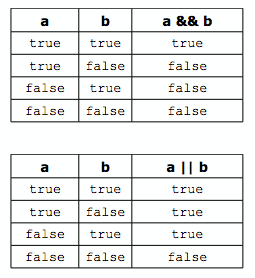# Erle Robotics C++ GitBook

## Operators

Conditionals (if statements) use two kinds of special operators: relational and logical. These are used to determine whether some condition is true or false.

###### Relational Operators

The relational operators are used to test a relation between two expressions:

Operator Meaning
> Greater than
>= Greater than or equal to
< Less than
<= Less than or equal to
== Equal to
!= Not equal to

They work the same as the arithmetic operators (e.g., a > b) but return a Boolean value of either true or false, indicating whether the relation tested for holds. (An expression that returns this kind of value is called a Boolean expression.) For example, if the variables x and y have been set to 6 and 2, respectively, then x > y returns true. Similarly, x < 5 returns false.

###### Logical Operators

The logical operators are often used to combine relational expressions into more complicated Boolean expressions:

Operator Meaning
&& and
double pipe or
! not

The and and or operators return true or false, according to the rules of logic:The not operator is a unary operator, taking only one argument and negating its value:Of course, Boolean variables can be used directly in these expressions, since they hold true and false values. In fact, any kind of value can be used in a Boolean expression due to a quirk C++ has: false is represented by a value of 0 and anything that is not 0 is true. So, “Hello, world!” is true, 2 is true, and any int variable holding a non-zero value is true. This means !x returns false and x && y returns true.